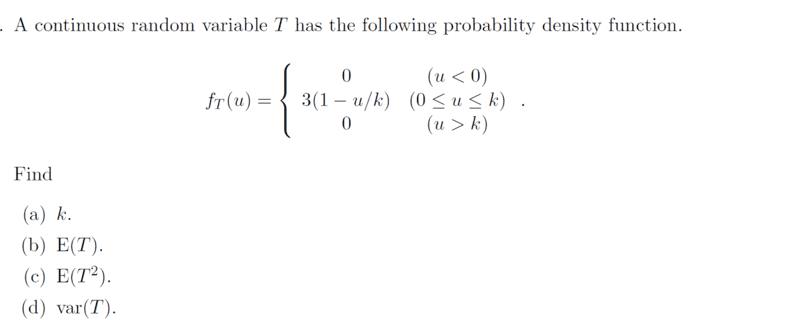# Solve this problem that involves a probability density function

• chwala
Where does the 6 in the variance come from? Shouldn't it be a 2?Where does the 6 in the variance come from? Shouldn't it be a 2?True...let me amend that.##E(T^2)=-\int_0^{\frac{2}{3}} u^2⋅(3-\dfrac{9}{2}u )du####=-\int_0^{\frac{2}{3}} (3u^2-\dfrac{9u^3}{2} )du=-\left[u^3-\dfrac{9u^4}{8} \right]####=-\left

#### chwala

Gold Member
Homework Statement
see attached
Relevant Equations
understanding of continous and discrete distribution
I am refreshing on this; ..after a long time...

Note that i do not have the solution to this problem.##f(u)= 3u-\dfrac{3u^2}{2k}## with limits ##0≤u≤k##

it follows that,

##3k - \dfrac{3k}{2}=1##

##\dfrac{3k}{2}=1##

##k=\dfrac {2}{3}##

For part (b),

##E(T)=\int_0^{\frac{2}{3}} u⋅(3-\dfrac{9}{2}u )du=\left[\dfrac{3}{2}×\dfrac{4}{9}-\dfrac{3}{2}×\dfrac{8}{27}\right]=\dfrac{6-4}{9}= \dfrac{2}{9}##

Ok let me know if that's correct...

Last edited:
Those both look OK to me. Do you know how to do the last two parts?

One minor  issue
chwala said:
##f(u)= 3u-\dfrac{3u^2}{2k}## with limits ##0≤u≤k##
This is bad notation, since ##f## it's already defined to be the pdf, not the cdf.

•chwala
Office_Shredder said:
Those both look OK to me. Do you know how to do the last two parts?

One minor  issue

This is bad notation, since ##f## it's already defined to be the pdf, not the cdf.
I will work on the last parts too...refreshing on this...should be fine ...Will post once done.

Office_Shredder said:
Those both look OK to me. Do you know how to do the last two parts?

One minor  issue

This is bad notation, since ##f## it's already defined to be the pdf, not the cdf.
I thought pdf is generally defined to be ##f_{u}## being the derivative of the continuous distribution ##f(u)## ...different books have different notations/language that I pretty find to be time waster...my focus is on the concept...

Which is the right notation? Thanks @Office_Shredder

Last edited:
chwala said:
Which is the right notation? Thanks @Office_Shredder
In my stats class we used ##f_{X}(x)## for the PDF and ##F_{X}(x)## for the CDF for a given continuous random variable ##X##. I think that this is fairly standard, because most books and lecture notes I found online used it.

•chwala
Office_Shredder said:
Those both look OK to me. Do you know how to do the last two parts?

One minor  issue

This is bad notation, since ##f## it's already defined to be the pdf, not the cdf.
##E(T^2)=\int_0^{\frac{2}{3}} u^2⋅(3-\dfrac{9}{2}u )du##

##=\int_0^{\frac{2}{3}} (3u^2-\dfrac{9u^3}{2} )du=\left[u^3-\dfrac{9u^4}{8} \right]##

##=\left[\dfrac{8}{27}-\dfrac{9}{8}×\dfrac{16}{81}\right]=\dfrac{8-6}{27}= \dfrac{2}{27}##

##⇒Var (T) = \dfrac{24-4}{81}=\dfrac{20}{81}##

...this part is easy and straightforward. Bingo!

Where does the 24 in the variance come from? Shouldn't it be a 6?

•chwala
Office_Shredder said:
Where does the 24 in the variance come from? Shouldn't it be a 6?
True...let me amend that.

chwala said:
##E(T^2)=\int_0^{\frac{2}{3}} u^2⋅(3-\dfrac{9}{2}u )du##

##=\int_0^{\frac{2}{3}} (3u^2-\dfrac{9u^3}{2} )du=\left[u^3-\dfrac{9u^4}{8} \right]##

##=\left[\dfrac{8}{27}-\dfrac{9}{8}×\dfrac{16}{81}\right]=\dfrac{8-6}{27}= \dfrac{2}{27}##

##⇒Var (T) = \dfrac{6-4}{81}=\dfrac{2}{81}##

...this part is easy and straightforward. Bingo!